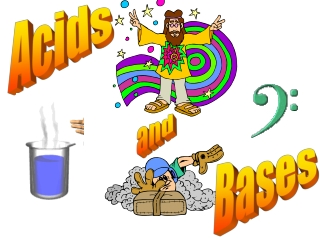DownloadDownload PresentationBases

Bases

Download PresentationBases

- - - - - - - - - - - - - - - - - - - - - - - - - - - E N D - - - - - - - - - - - - - - - - - - - - - - - - - - -
Presentation Transcript

1. Acids and Bases

2. Properties of acids and bases • Get 8 test tubes. Rinse all tubes well with water. Add acid to four tubes, base to the other four. • Touch a drop of base to your finger. Record the feel in the chart (on the next slide). Wash your hands with water. Repeat for acid. • Use a stirring rod, add base to the litmus and pH papers (for pH paper use a colour key to find a number). Record results. Repeat for acid. • Into the four base tubes add: a) two drops of phenolphthalein, b) 2 drops of bromothymol, c) a piece of Mg, d) a small scoop of baking soda. Record results. Repeat for acid. • Cleanup(wash tubes,pH/litmus paper in trash).

3. PROPERTIES *Usually, but not always BASE(aq) ACID(aq) Taste Bitter Sour “Feelings” Slippery Not slippery pH >7 <7 Litmus Blue Red Phenolphthalein Pink Clear & colorless Bromothymol *Blue *Yellow Reacts w/ Metal No Yes Electrolyte (conducts electricity in H2O) Yes Yes

4. pH • There are many ways to consider acids and bases. One of these is pH. Read pg. 368-70. • [H+] is critical in many chemical reactions. • A quick method of denoting [H+] is via pH. • By definition pH = –log [H+], [H+] = 10-pH • The pH scale, similar to the Richter scale, describes a wide range of values • An earthquake of “6” is 10 as violent as a “5” • Thus, the pH scale condenses possible values of [H+] to a 14 point scale (fig. 2, p370) • Also, it is easier to say pH=7 vs. [H+]=1x10–7

5. Calculations with pH Q: What is the pH if [H+]= 6.3 x 10–5? pH = –log [H+] (‘6.3’, ‘exp’ or ‘EE’, ‘5’, ‘+/-’, ‘log’, ‘+/-’) (‘-’, ‘log’, ‘6.3’, ‘exp’ or ‘EE’, ‘-’, ‘5’) Ans: 4.2 Q: What is the [H+] if pH = 7.4? [H+] = 10–pH mol/L (’10’, ‘xy’, ‘7.4’, ‘+/-’, ‘=‘) (’10’, ‘^’, ‘-’, ‘7.4’, ‘=‘) 3.98x10–8 M Try questions 2 and 6 (a-b) on page 375

6. Pg. 375 2 a) pH = –log [H+] = –log [1x10–8] = 8.0 b) pH = –log [H+] = –log [1x10–7] = 7.0 c) pH = –log [H+] = –log [2.5x10–6] = 5.60 d) pH = –log [H+] = –log [1.3x10–4] = 3.89 6 a) [H+] = 10–pH = 10–5.4 = 4 x 10–6 mol/L b) [H+] = 10–pH = 10–5.72 = 1.9 x 10–6 mol/L

7. Ionization + H H O H O Cl Cl H H H • O (e.g.H2SO4) was originally thought to cause acidic properties. Later, H was implicated, but it was still not clear why CH4 was neutral. • Arrhenius made the revolutionary suggestion that some solutions contain ions & that acids produce H3O+ (hydronium) ions in solution. Historical views on acids + + • The more recent Bronsted-Lowry concept is that acids are H+ (proton) donors and bases are proton acceptors

8. + + + H H O H O Cl Cl H H H • In this idea, the ionization of an acid by water is just one example of an acid-base reaction. The Bronsted-Lowry concept conjugate acid conjugate base acid base conjugate acid-base pairs • Acids and bases are identified based on whether they donate or accept H+. • “Conjugate” acids and bases are found on the products side of the equation. A conjugate base is the same as the starting acid minus H+.

9. Identify the acid, base, conjugate acid, conjugate base, and conjugate acid-base pairs: Practice problems HC2H3O2(aq) + H2O(l)  C2H3O2–(aq) + H3O+(aq) conjugate base conjugate acid acid base conjugate acid-base pairs OH–(aq) + HCO3–(aq)  CO32–(aq) + H2O(l) base acid conjugate base conjugate acid conjugate acid-base pairs • Reference: pg. 386 – 387 • Try Q18 (p389), Q 8 & 11 (p392): do as above

10. Answers: question 18 HF(aq) + SO32–(aq)  F–(aq) + HSO3–(aq) (a) conjugate base conjugate acid acid base conjugate acid-base pairs (b) CO32–(aq)+HC2H3O2(aq)C2H3O2–(aq)+HCO3–(aq) base acid conjugate base conjugate acid conjugate acid-base pairs (c) H3PO4(aq) + OCl–(aq)  H2PO4–(aq) + HOCl(aq) conjugate base conjugate acid acid base conjugate acid-base pairs

11. 8a) HCO3–(aq) + S2–(aq)  HS–(aq) + CO32–(aq) conjugate acid conjugate base acid base conjugate acid-base pairs 8b) H2CO3(aq) + OH–(aq)  HCO3–(aq) + H2O(l) acid base conjugate base conjugate acid conjugate acid-base pairs 11a) H3O+(aq) + HSO3–(aq)  H2O(l) + H2SO3(aq) conjugate base conjugate acid acid base conjugate acid-base pairs 11b) OH–(aq) + HSO3–(aq)  H2O(l) + SO32–(aq) conjugate acid conjugate base base acid conjugate acid-base pairs For more lessons, visit www.chalkbored.com